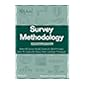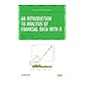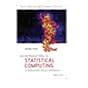Dr. S. R. Lasker Library Online Catalogue

Normal view

# An introduction to analysis of financial data with R / Ruey S. Tsay.

Material type:TextLanguage: English Publication details: c2013. Description: xiv, 390 p. : ill. ; 25 cmISBN: 9780470890813 (cloth)DDC classification: 519.5 LOC classification: HG106 | .T76 2013Online resources: WorldCat details | Ebook Fulltext
Contents:
TOC <p>Preface xiii <p>1 FINANCIAL DATA AND THEIR PROPERTIES 1 <p>1.1 Asset Returns 2 <p>1.2 Bond Yields and Prices 7 <p>1.3 Implied Volatility 10 <p>1.4 R Packages and Demonstrations 12 <p>1.4.1 Installation of R Packages 12 <p>1.4.2 The Quantmod Package 12 <p>1.4.3 Some Basic R Commands 16 <p>1.5 Examples of Financial Data 17 <p>1.6 Distributional Properties of Returns 20 <p>1.6.1 Review of Statistical Distributions and Their Moments20 <p>1.7 Visualization of Financial Data 27 <p>1.8 Some Statistical Distributions 32 <p>1.8.1 Normal Distribution 32 <p>1.8.2 Lognormal Distribution 32 <p>1.8.3 Stable Distribution 33 <p>1.8.4 Scale Mixture of Normal Distributions 33 <p>1.8.5 Multivariate Returns 34 <p>Exercises 36 <p>References 37 <p>2 LINEAR MODELS FOR FINANCIAL TIME SERIES 39 <p>2.1 Stationarity 40 <p>2.2 Correlation and Autocorrelation Function 43 <p>2.3 White Noise and Linear Time Series 50 <p>2.4 Simple Autoregressive Models 51 <p>2.4.1 Properties of AR Models 52 <p>2.4.2 Identifying AR Models in Practice 60 <p>2.4.3 Goodness of Fit 67 <p>2.4.4 Forecasting 67 <p>2.5 Simple Moving Average Models 69 <p>2.5.1 Properties of MA Models 72 <p>2.5.2 Identifying MA Order 73 <p>2.5.3 Estimation 74 <p>2.5.4 Forecasting Using MA Models 75 <p>2.6 Simple ARMA Models 78 <p>2.6.1 Properties of ARMA(1,1) Models 79 <p>2.6.2 General ARMA Models 80 <p>2.6.3 Identifying ARMA Models 81 <p>2.6.4 Forecasting Using an ARMA Model 84 <p>2.6.5 Three Model Representations for an ARMA Model 84 <p>2.7 Unit-Root Nonstationarity 86 <p>2.7.1 Random Walk 86 <p>2.7.2 Random Walk with Drift 88 <p>2.7.3 Trend-Stationary Time Series 90 <p>2.7.4 General Unit-Root Nonstationary Models 91 <p>2.7.5 Unit-Root Test 91 <p>2.8 Exponential Smoothing 96 <p>2.9 Seasonal Models 98 <p>2.9.1 Seasonal Differencing 99 <p>2.9.2 Multiplicative Seasonal Models 101 <p>2.9.3 Seasonal Dummy Variable 107 <p>2.10 Regression Models with Time Series Errors 110 <p>2.11 Long-Memory Models 117 <p>2.12 Model Comparison and Averaging 120 <p>2.12.1 In-sample Comparison 120 <p>2.12.2 Out-of-sample Comparison 121 <p>2.12.3 Model Averaging 125 <p>Exercises 125 <p>References 127 <p>3 CASE STUDIES OF LINEAR TIME SERIES 128 <p>3.1 Weekly Regular Gasoline Price 129 <p>3.1.1 Pure Time Series Model 130 <p>3.1.2 Use of Crude Oil Prices 133 <p>3.1.3 Use of Lagged Crude Oil Prices 134 <p>3.1.4 Out-of-Sample Predictions 135 <p>3.2 Global Temperature Anomalies 140 <p>3.2.1 Unit-Root Stationarity 141 <p>3.2.2 Trend-Nonstationarity 145 <p>3.2.3 Model Comparison 148 <p>3.2.4 Long-Term Prediction 150 <p>3.2.5 Discussion 153 <p>3.3 US Monthly Unemployment Rates 157 <p>3.3.1 Univariate Time Series Models 157 <p>3.3.2 An Alternative Model 161 <p>3.3.3 Model Comparison 165 <p>3.3.4 Use of Initial Jobless Claims 165 <p>3.3.5 Comparison 173 <p>Exercises 174 <p>References 175 <p>4 ASSET VOLATILITY AND VOLATILITY MODELS 176 <p>4.1 Characteristics of Volatility 177 <p>4.2 Structure of a Model 178 <p>4.3 Model Building 181 <p>4.4 Testing for ARCH Effect 182 <p>4.5 The ARCH Model 185 <p>4.5.1 Properties of ARCH Models 186 <p>4.5.2 Advantages and Weaknesses of ARCH Models 187 <p>4.5.3 Building an ARCH Model 188 <p>4.5.4 Some Examples 193 <p>4.6 The GARCH Model 199 <p>4.6.1 An Illustrative Example 201 <p>4.6.2 Forecasting Evaluation 210 <p>4.6.3 A Two-Pass Estimation Method 210 <p>4.7 The Integrated GARCH Model 211 <p>4.8 The GARCH-M Model 213 <p>4.9 The Exponential Garch Model 215 <p>4.9.1 An Illustrative Example 217 <p>4.9.2 An Alternative Model Form 218 <p>4.9.3 Second Example 218 <p>4.9.4 Forecasting Using an EGARCH Model 220 <p>4.10 The Threshold Garch Model 222 <p>4.11 Asymmetric Power ARCH Models 224 <p>4.12 Nonsymmetric GARCH Model 226 <p>4.13 The Stochastic Volatility Model 228 <p>4.14 Long-Memory Stochastic Volatility Models 230 <p>4.15 Alternative Approaches 232 <p>4.15.1 Use of High Frequency Data 232 <p>4.15.2 Use of Daily Open, High, Low, and Close Prices 235 <p>Exercises 239 <p>References 241 <p>5 APPLICATIONS OF VOLATILITY MODELS 243 <p>5.1 Garch Volatility Term Structure 244 <p>5.1.1 Term Structure 246 <p>5.2 Option Pricing and Hedging 248 <p>5.3 Time-Varying Correlations and Betas 251 <p>5.3.1 Time-Varying Betas 256 <p>5.4 Minimum Variance Portfolios 259 <p>5.5 Prediction 263 <p>Exercises 271 <p>References 272 <p>6 HIGH FREQUENCY FINANCIAL DATA 274 <p>6.1 Nonsynchronous Trading 275 <p>6.2 Bid Ask Spread of Trading Prices 279 <p>6.3 Empirical Characteristics of Trading Data 282 <p>6.4 Models for Price Changes 285 <p>6.4.1 Ordered Probit Model 288 <p>6.4.2 A Decomposition Model 293 <p>6.5 Duration Models 298 <p>6.5.1 Diurnal Component 299 <p>6.5.2 The ACD Model 301 <p>6.5.3 Estimation 303 <p>6.6 Realized Volatility 308 <p>6.6.1 Handling Microstructure Noises 313 <p>6.6.2 Discussion 317 <p>Appendix A: Some Probability Distributions 320 <p>Appendix B: Hazard Function 323 <p>Exercises 324 <p>References 325 <p>7 VALUE AT RISK 327 <p>7.1 Risk Measure and Coherence 328 <p>7.1.1 Value at Risk (VaR) 329 <p>7.1.2 Expected Shortfall 334 <p>7.2 Remarks on Calculating Risk Measures 336 <p>7.3 Riskmetrics 337 <p>7.3.1 Discussion 342 <p>7.3.2 Multiple Positions 343 <p>7.4 An Econometric Approach 345 <p>7.4.1 Multiple Periods 348 <p>7.5 Quantile Estimation 352 <p>7.5.1 Quantile and Order Statistics 353 <p>7.5.2 Quantile Regression 354 <p>7.6 Extreme Value Theory 358 <p>7.6.1 Review of Extreme Value Theory 358 <p>7.6.2 Empirical Estimation 361 <p>7.6.3 Application to Stock Returns 363 <p>7.7 An Extreme Value Approach to Var 368 <p>7.7.1 Discussion 370 <p>7.7.2 Multiperiod VaR 371 <p>7.7.3 Return Level 371 <p>7.8 Peaks Over Thresholds 372 <p>7.8.1 Statistical Theory 373 <p>7.8.2 Mean Excess Function 374 <p>7.8.3 Estimation 376 <p>7.8.4 An Alternative Parameterization 378 <p>7.9 The Stationary Loss Processes 381 <p>Exercises 383 <p>References 384 <p>Index 387
Summary: "An Introduction to Analysis of Financial Data with R explores basic concepts of visualization of financial data. Through a fundamental balance between theory and applications, the book supplies readers with an accessible approach to financial econometric models and their applications to real-world empirical research. The author supplies a hands-on introduction to the analysis of financial data using the freely available R software package and case studies to illustrate actual implementations of the discussed methods. The book begins with the basics of financial data, discussing their summary statistics and related visualization methods. Subsequent chapters explore basic time series analysis and simple econometric models for business, finance, and economics as well as related topics including: Linear time series analysis, with coverage of exponential smoothing for forecasting and methods for model comparison; Different approaches to calculating asset volatility and various volatility models; High-frequency financial data and simple models for price changes, trading intensity, and realized volatility; Quantitative methods for risk management, including value at risk and conditional value at risk; Econometric and statistical methods for risk assessment based on extreme value theory and quantile regression. Throughout the book, the visual nature of the topic is showcased through graphical representations in R, and two detailed case studies demonstrate the relevance of statistics in finance. A related website features additional data sets and R scripts so readers can create their own simulations and test their comprehension of the presented techniques"--Back cover.
Tags from this library: No tags from this library for this title.
Star ratingsAverage rating: 0.0 (0 votes)
Holdings
Item type Current library Collection Call number Copy number Status Date due Barcode Item holdsE-Book
E-book
Non-fiction 519.5 TSI 2013 (Browse shelf(Opens below)) Not for loanText
Reserve Section
Non-fiction 519.5 TSI 2013 (Browse shelf(Opens below)) C-1 Not For Loan 26669Text
Circulation Section
Non-fiction 519.5 TSI 2013 (Browse shelf(Opens below)) C-2 Available 26670
Total holds: 0
##### Browsing EWU Library shelves, Shelving location: E-book Close shelf browser (Hides shelf browser)519.5 STA 2012 Statistical methods in healthcare / 519.5 SUR 2009 Survey methodology / 519.5 TRP 1982 Probability and statistics with reliability, queuing, and computer science applications / 519.5 TSI 2013 An introduction to analysis of financial data with R / 519.5 WEI 2012 Introductory statistics. 519.50113 VOI 2014 An introduction to statistical computing : 519.502 BEC 2012 Competing risks and multistate models with R /

Includes bibliographical references and index.

TOC <p>Preface xiii <p>1 FINANCIAL DATA AND THEIR PROPERTIES 1 <p>1.1 Asset Returns 2 <p>1.2 Bond Yields and Prices 7 <p>1.3 Implied Volatility 10 <p>1.4 R Packages and Demonstrations 12 <p>1.4.1 Installation of R Packages 12 <p>1.4.2 The Quantmod Package 12 <p>1.4.3 Some Basic R Commands 16 <p>1.5 Examples of Financial Data 17 <p>1.6 Distributional Properties of Returns 20 <p>1.6.1 Review of Statistical Distributions and Their Moments20 <p>1.7 Visualization of Financial Data 27 <p>1.8 Some Statistical Distributions 32 <p>1.8.1 Normal Distribution 32 <p>1.8.2 Lognormal Distribution 32 <p>1.8.3 Stable Distribution 33 <p>1.8.4 Scale Mixture of Normal Distributions 33 <p>1.8.5 Multivariate Returns 34 <p>Exercises 36 <p>References 37 <p>2 LINEAR MODELS FOR FINANCIAL TIME SERIES 39 <p>2.1 Stationarity 40 <p>2.2 Correlation and Autocorrelation Function 43 <p>2.3 White Noise and Linear Time Series 50 <p>2.4 Simple Autoregressive Models 51 <p>2.4.1 Properties of AR Models 52 <p>2.4.2 Identifying AR Models in Practice 60 <p>2.4.3 Goodness of Fit 67 <p>2.4.4 Forecasting 67 <p>2.5 Simple Moving Average Models 69 <p>2.5.1 Properties of MA Models 72 <p>2.5.2 Identifying MA Order 73 <p>2.5.3 Estimation 74 <p>2.5.4 Forecasting Using MA Models 75 <p>2.6 Simple ARMA Models 78 <p>2.6.1 Properties of ARMA(1,1) Models 79 <p>2.6.2 General ARMA Models 80 <p>2.6.3 Identifying ARMA Models 81 <p>2.6.4 Forecasting Using an ARMA Model 84 <p>2.6.5 Three Model Representations for an ARMA Model 84 <p>2.7 Unit-Root Nonstationarity 86 <p>2.7.1 Random Walk 86 <p>2.7.2 Random Walk with Drift 88 <p>2.7.3 Trend-Stationary Time Series 90 <p>2.7.4 General Unit-Root Nonstationary Models 91 <p>2.7.5 Unit-Root Test 91 <p>2.8 Exponential Smoothing 96 <p>2.9 Seasonal Models 98 <p>2.9.1 Seasonal Differencing 99 <p>2.9.2 Multiplicative Seasonal Models 101 <p>2.9.3 Seasonal Dummy Variable 107 <p>2.10 Regression Models with Time Series Errors 110 <p>2.11 Long-Memory Models 117 <p>2.12 Model Comparison and Averaging 120 <p>2.12.1 In-sample Comparison 120 <p>2.12.2 Out-of-sample Comparison 121 <p>2.12.3 Model Averaging 125 <p>Exercises 125 <p>References 127 <p>3 CASE STUDIES OF LINEAR TIME SERIES 128 <p>3.1 Weekly Regular Gasoline Price 129 <p>3.1.1 Pure Time Series Model 130 <p>3.1.2 Use of Crude Oil Prices 133 <p>3.1.3 Use of Lagged Crude Oil Prices 134 <p>3.1.4 Out-of-Sample Predictions 135 <p>3.2 Global Temperature Anomalies 140 <p>3.2.1 Unit-Root Stationarity 141 <p>3.2.2 Trend-Nonstationarity 145 <p>3.2.3 Model Comparison 148 <p>3.2.4 Long-Term Prediction 150 <p>3.2.5 Discussion 153 <p>3.3 US Monthly Unemployment Rates 157 <p>3.3.1 Univariate Time Series Models 157 <p>3.3.2 An Alternative Model 161 <p>3.3.3 Model Comparison 165 <p>3.3.4 Use of Initial Jobless Claims 165 <p>3.3.5 Comparison 173 <p>Exercises 174 <p>References 175 <p>4 ASSET VOLATILITY AND VOLATILITY MODELS 176 <p>4.1 Characteristics of Volatility 177 <p>4.2 Structure of a Model 178 <p>4.3 Model Building 181 <p>4.4 Testing for ARCH Effect 182 <p>4.5 The ARCH Model 185 <p>4.5.1 Properties of ARCH Models 186 <p>4.5.2 Advantages and Weaknesses of ARCH Models 187 <p>4.5.3 Building an ARCH Model 188 <p>4.5.4 Some Examples 193 <p>4.6 The GARCH Model 199 <p>4.6.1 An Illustrative Example 201 <p>4.6.2 Forecasting Evaluation 210 <p>4.6.3 A Two-Pass Estimation Method 210 <p>4.7 The Integrated GARCH Model 211 <p>4.8 The GARCH-M Model 213 <p>4.9 The Exponential Garch Model 215 <p>4.9.1 An Illustrative Example 217 <p>4.9.2 An Alternative Model Form 218 <p>4.9.3 Second Example 218 <p>4.9.4 Forecasting Using an EGARCH Model 220 <p>4.10 The Threshold Garch Model 222 <p>4.11 Asymmetric Power ARCH Models 224 <p>4.12 Nonsymmetric GARCH Model 226 <p>4.13 The Stochastic Volatility Model 228 <p>4.14 Long-Memory Stochastic Volatility Models 230 <p>4.15 Alternative Approaches 232 <p>4.15.1 Use of High Frequency Data 232 <p>4.15.2 Use of Daily Open, High, Low, and Close Prices 235 <p>Exercises 239 <p>References 241 <p>5 APPLICATIONS OF VOLATILITY MODELS 243 <p>5.1 Garch Volatility Term Structure 244 <p>5.1.1 Term Structure 246 <p>5.2 Option Pricing and Hedging 248 <p>5.3 Time-Varying Correlations and Betas 251 <p>5.3.1 Time-Varying Betas 256 <p>5.4 Minimum Variance Portfolios 259 <p>5.5 Prediction 263 <p>Exercises 271 <p>References 272 <p>6 HIGH FREQUENCY FINANCIAL DATA 274 <p>6.1 Nonsynchronous Trading 275 <p>6.2 Bid Ask Spread of Trading Prices 279 <p>6.3 Empirical Characteristics of Trading Data 282 <p>6.4 Models for Price Changes 285 <p>6.4.1 Ordered Probit Model 288 <p>6.4.2 A Decomposition Model 293 <p>6.5 Duration Models 298 <p>6.5.1 Diurnal Component 299 <p>6.5.2 The ACD Model 301 <p>6.5.3 Estimation 303 <p>6.6 Realized Volatility 308 <p>6.6.1 Handling Microstructure Noises 313 <p>6.6.2 Discussion 317 <p>Appendix A: Some Probability Distributions 320 <p>Appendix B: Hazard Function 323 <p>Exercises 324 <p>References 325 <p>7 VALUE AT RISK 327 <p>7.1 Risk Measure and Coherence 328 <p>7.1.1 Value at Risk (VaR) 329 <p>7.1.2 Expected Shortfall 334 <p>7.2 Remarks on Calculating Risk Measures 336 <p>7.3 Riskmetrics 337 <p>7.3.1 Discussion 342 <p>7.3.2 Multiple Positions 343 <p>7.4 An Econometric Approach 345 <p>7.4.1 Multiple Periods 348 <p>7.5 Quantile Estimation 352 <p>7.5.1 Quantile and Order Statistics 353 <p>7.5.2 Quantile Regression 354 <p>7.6 Extreme Value Theory 358 <p>7.6.1 Review of Extreme Value Theory 358 <p>7.6.2 Empirical Estimation 361 <p>7.6.3 Application to Stock Returns 363 <p>7.7 An Extreme Value Approach to Var 368 <p>7.7.1 Discussion 370 <p>7.7.2 Multiperiod VaR 371 <p>7.7.3 Return Level 371 <p>7.8 Peaks Over Thresholds 372 <p>7.8.1 Statistical Theory 373 <p>7.8.2 Mean Excess Function 374 <p>7.8.3 Estimation 376 <p>7.8.4 An Alternative Parameterization 378 <p>7.9 The Stationary Loss Processes 381 <p>Exercises 383 <p>References 384 <p>Index 387

"An Introduction to Analysis of Financial Data with R explores basic concepts of visualization of financial data. Through a fundamental balance between theory and applications, the book supplies readers with an accessible approach to financial econometric models and their applications to real-world empirical research. The author supplies a hands-on introduction to the analysis of financial data using the freely available R software package and case studies to illustrate actual implementations of the discussed methods. The book begins with the basics of financial data, discussing their summary statistics and related visualization methods. Subsequent chapters explore basic time series analysis and simple econometric models for business, finance, and economics as well as related topics including: Linear time series analysis, with coverage of exponential smoothing for forecasting and methods for model comparison; Different approaches to calculating asset volatility and various volatility models; High-frequency financial data and simple models for price changes, trading intensity, and realized volatility; Quantitative methods for risk management, including value at risk and conditional value at risk; Econometric and statistical methods for risk assessment based on extreme value theory and quantile regression. Throughout the book, the visual nature of the topic is showcased through graphical representations in R, and two detailed case studies demonstrate the relevance of statistics in finance. A related website features additional data sets and R scripts so readers can create their own simulations and test their comprehension of the presented techniques"--Back cover.

AS

Tahur Ahmed

There are no comments on this title.